## 3rd UK User Group meeting: Panel Data estimators

James W. Hardin
London Users Group meeting
6 June 1997

Outline

1. Panel data
2. Estimators
3. Identifiability issues
4. Monte Carlo Simulation
5. Summary

References

1991
J.M. Neuhaus, J.D. Kalbfleisch, and W.W. Hauck
A Comparison of Cluster-Specific and Population Averaged Approaches for Analyzing Correlated Binary Data
International Statistical Review 59, 25-35.

Comparison of PA and SS models. Authors present two approaches to comparing these models. Good in combination with the Zeger/Liang/Albert paper.

References

1996
J.F. Pendergast, S.J. Gange, M.A. Newton, M.J. Lindstrom, M. Palta, and M.R. Fisher
A Survey of Methods for Analyzing Clustered Binary Response Data
International Statistical Review 64, 89-118.

Survey paper with canonical list of proposed methods. Includes nice exposition on comparing the methods and a very good long reference list.

References

1992
J.M. Neuhaus
Statistical methods for longitudinal and clustered designs
with binary responses
Statistical Methods in Medical Research 1, 249-273.

Survey paper which covers not only the PA and SS models, but also covers the transitional models, response conditional models, and some hybrid models. This paper also presents a data analysis example from a longitudinal study of AIDS behaviors among men in San Francisco which I will use in order to present the types of hypotheses addressed by the various panel estimators.

References

1988
G. Chamberlain
Analysis of Covariance with Qualitative Data
Review of Economic Studies 225-238.

Comparison of fixed (including conditional) effects and random effects (focusing on PA models).

References

1988
S.L. Zeger, K.-Y. Liang, and P.S. Albert
Models for Longitudinal Data: A Generalized Estimating Equation Approach
Biometrics 44, 1049-1060.

Comparison of SS and PA models for longitudinal data. An alternative comparison here from the presentation in the
Neuhaus paper.

References

1986
K.-Y. Liang and S.L. Zeger
Longitudinal data analysis using generalized linear models
Biometrika 73, 13-22.

This paper was the introduction of the GEE PA model that is in Stata (xtgee).

Panel Data

In a panel dataset, we have observations for our dependent variable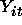such that the observations with common value for i are believed to be correlated. The i subscript is sometimes referred to as the individual, panel, subject, cluster, or group. The t subscript denotes the observation for the particular panel. There are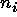observations in the general unbalanced case. The t subscript is called the replication, time, or repeated measure.

Various authors refer to longitudinal data, cross sectional
data, panel data, and cross-sectional time-series.

Estimators

There are two sources of variability from which we might build an estimator. There is the variability within (fixed effects) a cluster and there is the variability between the clusters.

Fixed Effects Estimators

To model fixed effects, one transforms the estimating equation in order to get rid of the fixed effects.

Random Effects Estimators

There are two obvious ways to approach building a random effects estimator. One may first assume that: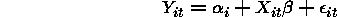where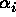is a random value from some distribution F. Alternatively, one may assume that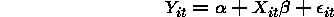and impose some restrictions on the covariance ofRandom Effects Estimators

In more general terms we can write the model in terms of link and variance functions as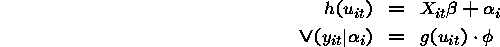where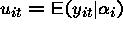or we may assume that with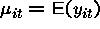we have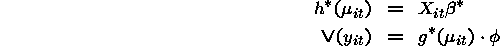Random Effects Estimators

When are the two approaches the same?

They are the same if all of the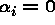or when the link function h is the identity. This is because while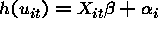, it is not in general true that the same link function will have the property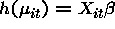.

Note that the two approaches are the same for linear regression which uses the identity link. They are not the same for logistic or probit models that we examine later.

Random Effects Estimators (logit)

The two approaches for logit are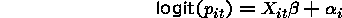or alternatively, we may look at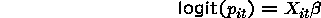along with appropriate assumptions on the covariance of the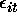terms (nuisance parameters) and where we assume that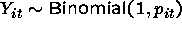.

Multilevel models

There are also hybrid models that will estimate the probability that Y=1 averaged over the observations with the same covariate patterns. One method for doing this is Goldstein's multilevel models. These models at their simplest level are random effects models, but allow the researcher more flexibility in modeling the outcome.

Other Models

There are also other types of models one can use for analyzing the panel data. The first is called the transitional model and models the probability distribution of the outcome at time t,as a function of the covariates at time t,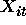, and the individual’s outcome history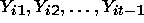.

Another model is called the response conditional model which accomodates correlation by modelling the response probability for each individual in the panel as a function of covariates for that individual and the responses for all individuals in that cluster.

Problems with SS Models

1. Problems fitting cluster level variables
2. Must have more than one observation per panel

Problems with PA Models

1. Ignores information leading to coefficient attenuation

Comparison of SS and PA coefficients

Imagine a study where the dependent variable is whether a student performs acceptably on a standardized test. There are several students under the direction of each teacher in the study. One of the covariates is whether the student’s instructor assigns to the individual student Stata in the classroom for teaching purposes.

Usually, one would consider that the instructor would either use Stata or not use it in teaching all of the teacher's students. However, imagine that an instructor is free to assign Stata to some of the students in the classroom but not to all of the students. So, the use of Stata is not a cluster level variable.

Interpretation of the coefficient for the SS model

The SS model now allows direct observation and estimation of the average log odds ratio effect of the change in using Stata to teach upon exam performance. Mathematically, we collapse across students after we take the difference in log odds at time points where the instructor did and did not use Stata in the classroom. The coefficient then represents the common log odds ratio for passing the exam of the Stata effect across students.

Interpretation of the coefficient for the PA model

The PA coefficient, mathematically, first averages to find the mean risk and then computes the log odds. The PA model ignores the fact that the effect of the change in using Stata for an instructor had been measured, and persist in estimating only the odds ratio between Stata and non-Stata instructors. Instructors who changed would appear in both groups.

Now imagine, that there really are not any instructors that assign Stata to a subset of the class so that Stata use is really a cluster level variable.

One cannot directly observe a change in utilizing Stata. The PA model measures the log odds ratio between the two groups of instructors, whereas the SS model is supposed to report the effect of the change in the instructor's usage of Stata. However, no such change was measured, so the interpretation is entirely model-based as it is a type of extrapolation with no data to check the validity of the extrapolation. Note that the conditional likelihood approach for this same model won't allow estimation of the Stata effect.

Problems with Conditional models

1. Can not fit cluster level variables
2. Need to check for cluster level collinearity in each cluster

Note that for the logit estimator, the unconditional
fixed-effects estimator is inconsistent, but the conditional
estimator is consistent. Let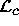denote the conditional log-likelihood below.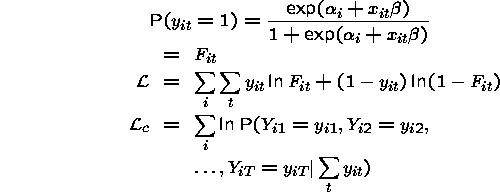So, the conditional likelihood is conditioned on the number of ones in the set (panel). Consider an example where there are a large number of panels each with two time period observations. The unconditional likelihood is given by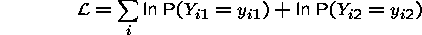The observations are independent so that the likelihood
function is the product of the probabilities (we show above the log-likelihood). Note that for each pair of observations, we have the possibilities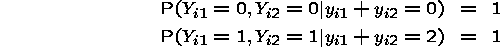The ith term offor either of these outcomes is just 1. The log of that is zero, so that either of these outcomes contribute nothing to the log-likelihood.

Now, suppose that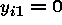and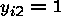so that we have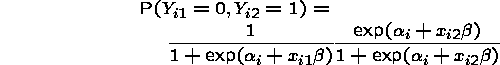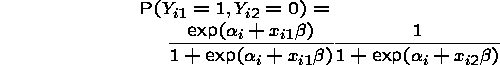which gives that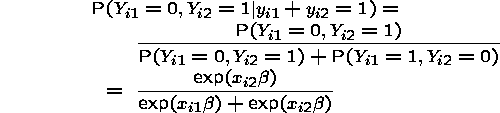which is free of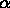.

Monte Carlo Simulations

There are two simulations that we ran both generating SS random effects data.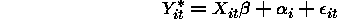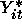is an unobserved latent variable.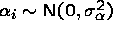is the random effect.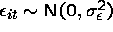is the error term.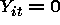if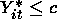where c is some cutoff value.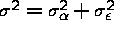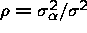Estimators

1. Probit
2. Probit with robust standard errors
3. Maximum likelihood SS random effects probit
4. GEE PA probit (exchangeable correlation)
5. GEE PA probit (exchangeable correlation) with robust standard errors

Other Estimators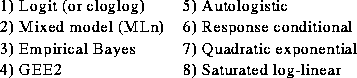Simulation 1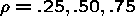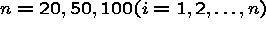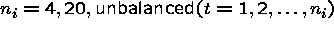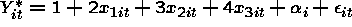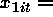constant within panel (cluster level variable).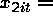constant across panels (within time)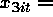random within and across panels.
r = 1000 is the number of simulations for a given model.

Simulation 2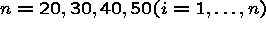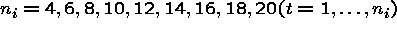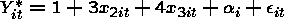constant across panels (within time)random within and across panels.
r = 500 is the number of simulations for a given model.

The main differences for the second simulation were the removal of the cluster level variable and the focus on smaller datasets.

Random Effects Likelihood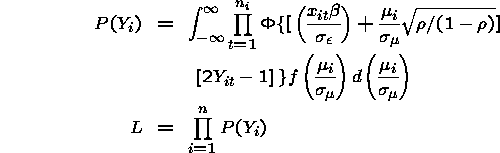Problems with SS Random Effects Probit

1. Problems fitting cluster level variables

Simulation Results

Probit

1. Coverage probability below nominal level.
2. Derived test statistics not normally distributed even at very large sample sizes. As cluster size grows or as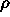becomes larger, the estimated standard errors are too small.

Simulation Results

Probit

The probit estimator differed little from the SS-RE model in terms of RMSE: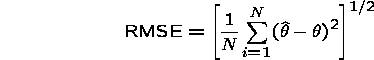However, misleading results will result if one uses the reported standard errors in hypothesis tests.

Simulation Results

Probit with robust standard errors

1. Coverage probability near nominal level.
2. Derived test statistics normally distributed when overall sample sizes are larger than 1000 andis small.

Simulation Results

SS Random Effects Probit

1. Very good for small samples with low correlation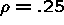.
2. Numeric problems with the quadrature calculations
when either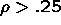orbecomes large (more than 10).

Simulation Results

SS Random Effects Probit

The major computational problem with the SS Random Effects Probit model is the need to evaluate the integral using quadrature. It is for these numeric reasons that this estimator did not perform better. However, it dominated the other estimators for small values ofand. One gains substantial improvement by increasing the number of Hermite points to about 8 to 10, but not much improvement after that. Guilkey and Murphy found it necessary to increase this to 16 for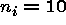and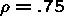to obtain good performance.

Simulation Results

SS Random Effects Probit

For Simulation 1, where for the cluster level variable, the SS RE Probit estimator had lower than nominal coverage and a much larger standard error than the PA Estimator. Whenwas small (4), the coverage was close to nominal though the RMSE was larger than for the population averaged approach.

Estimated standard errors are too small whenorget large due to numerical problems of estimating the integral (not because the model is faulty).

Simulation Results

PA Random Effects Probit

1. Coverage probability near nominal level only for.
2. Derived test statistics normally distributed when overall sample sizes are larger than 1000 andis small.
3. Coefficient estimates smaller than SS model.

Simulation Results

PA Random Effects Probit

Coefficients were smaller than for the SS model as theory dictates. The standard errors were too small, but coverage is close to nominal level for small cluster size even when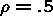, but not close to nominal coverage when.

Simulation Results

PA Random Effects Probit with robust standard errors

1. Coverage probability near nominal when n>30.
2. Derived test statistics normally distributed when overall sample sizes are larger than 1000 andis small.

Simulation Results

PA Random Effects Probit with robust standard errors

Coefficients were smaller than for the SS model as theory dictates. The standard errors are of correct size and the coverage is close to nominal size for all sample sizes and values of.

Summary

Difference in PA and SS models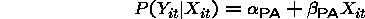with appropriate assumptions concerning the covariance of.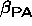measures the change in proportion with Y=1 for a unit increase in X. Does not take advantage of repeated measurements on each study subject and the fact that the effects of the covariate changes within subjects on the response are directly observable. This model is most appropriate for cluster level variables.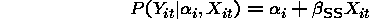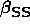measures the change in probability of response with covariate X for individuals in each of the underlying risk groups described by. Not appropriate for cluster level variables since this effect is not directly observable.

Problems with Conditional models

1. Can not fit cluster level variables
2. Need to check for cluster level collinearity in
each cluster